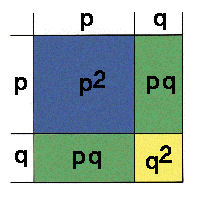Derivation of the Hardy-Weinberg Theorem:
three methods

In a monoecious species, for a single locus with two alleles A & a:
Let frequency of A allele f(A) = p
Let frequency of a allele f(a) = q
Let allele frequenices in males & females be equal.
What are the expected genotype frequencies?

What are the probabilities of obtaining various gametic combinations?
prob. of A from male & A from female:     pm x pf = p2
prob. of a from male & a from female:      qm x q = q2
prob. of A from male & a from female,
or a from male & A from female:     (pm x qf ) + (pf x qm ) = 2pq

What is the result of the binomial expansion of p & q in males & females

(pm + qm) (pf + qf) = p2 + 2pq + q2

What are the areas of the four boxes in a Punnet Square?By all three methods:    f(AA) = p2         f(Aa) = 2pq        f(aa) = q2

All text material © 2004 by Steven M. Carr